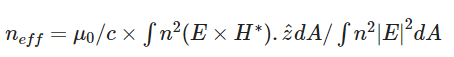## Photonics

•Guanhui Wang
Ansys Employee
Is there any easy two calculate effective refractive index for an structure?nfor example, imagine we have a layer of gold that is containing randomly sized and randomly dispersed cavities inside it, so we can define an effective refractive index for whole medium.n
•Guanhui Wang
Ansys Employee
Effective index can be defined for a mode. Depending on the mode profile/frequency, you can obtain different effective index for the modes. If the waveguide cross section is uniform, you can use MODE/FDE or port object in FDTD to calculate the effective index of the mode.nAnother option would be to find the field profile of the resonant mode, and then use the equation below to calculate the effective index:nwhere n is the material index, and z is the unit vector perpendicular to the surface of the cavity. The calculations will be similar to the scripts that we have for mode volume:nhttps://kb.lumerical.com/layout_analysis_model_volume.html 69n